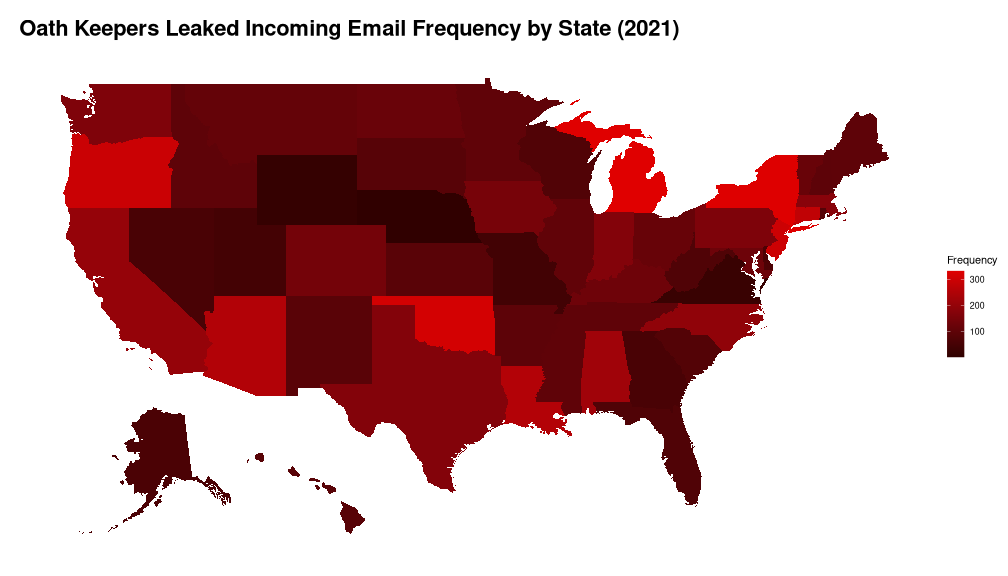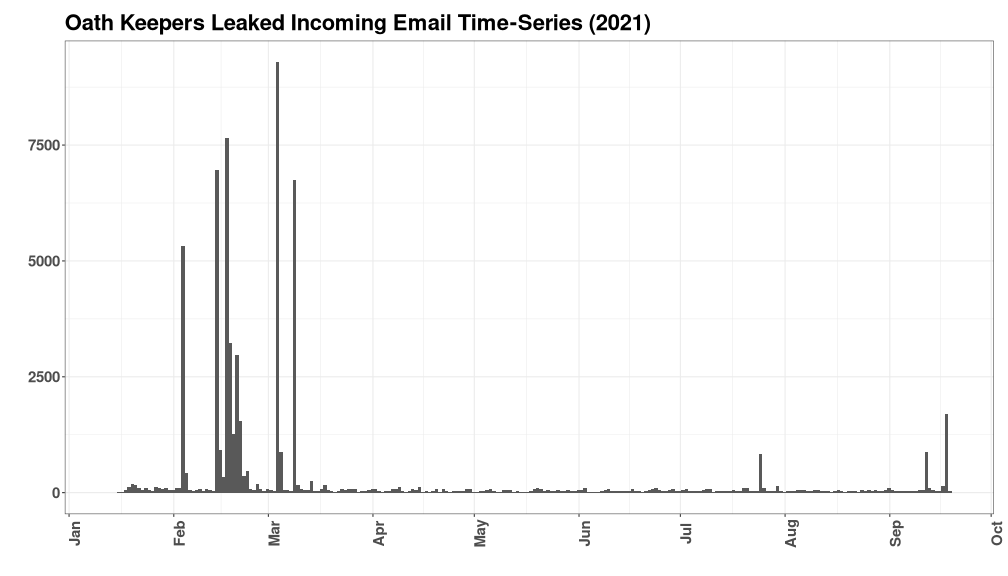# Oath Keepers Leaked Incoming Emails State Frequency Map

https://www.theguardian.com/us-news/2021/oct/01/capitol-attack-oath-keepers-far-right-militia-group

The recent Oath Keepers leak had emails separated by state code (xx@oathkeepers.org). The frequency of emails sent from people to the various addresses can be obtained and converted to CSV with the following command from the MBOX file directory:

```#!/bin/bash

grep -E "^From: " OathKeepers/Oath\ Keepers.sbd/* | \
cut -d ':' -f1 | \
cut -d '/' -f3 | \
grep -E "^[a-z][a-z]\$" | \
sort | uniq -c | \
sed -r "s/^[ ]+//g" | \
tr ' ' ',' \
> ok_state_freq.csv
```

These emails represent people attempting to sign up who vary in background, but also a few hate mails and a bunch of spam. The results are not very conclusive about anything other than which email addresses are most frequently used.

For time series data, this command can parse the dates effectively (as parsed off of sender's email service, not by received date). This uses the UNIX “date” command to parse, which is very versatile and accepts many forms of input.

```#!/bin/bash

grep -E "^Date: " OathKeepers/Oath\ Keepers.sbd/* | \
cut -d ':' -f3- | \
sed -r "s/(<br>|Subject: ).*\$//g" | \
sed -r "s/  .*\$//g" | \
sed -r "s/^[ \t]//g" | \
sed -r "s/ (at|from) //g" | \
sed -r "s/ 2021([0-9])/ 2021 \1/g" | \
sed "s/2021, /2021 /g" | \
sed -r "s/[\t=].*\$//g" | \
sed -r "s/ ([0-9][0-9])(st|nd|rd|th) / \1 /g" | \
sed -r "s/ ([0-9][0-9]?)am/ \1:00/g" | \
xargs -I{} date -d {} | \
sed -r "s/ ([A-Z][A-Z]T) ([0-9]{4})\$/ \2,\1/g" \
> ok_email_times.txt
```

Which only yields one error:

``````date: invalid date ‘Thursday February 2021 10:00’
``````

Since it has no actual date value in the field and is largely gibberish. The full value provided via whomever's email client:

``````\$ grep "Thursday February 2021" *
contact:Date: Thursday February 2021 from 10am=C2=A0 to 12pm (Central European Ti=
``````

The drop off in the time-series graph can be explained scrolling down a bit on this archived version of the site. Just subtract the hysteria, hyperbole, paranoia, and fear.

https://web.archive.org/web/20210401042418/https://oathkeepers.org/

## Load Data Into R

``````library(ggplot2)
library(mapproj)
library(scales)
library(fiftystater)
``````
``````state_codes <- read.csv("shared/state_codes.csv")
``````
``````ok_state_freq <- read.csv("ok_state_freq.csv", header=FALSE, col.names=c("Frequency", "State.Code"))
``````
``````ok_state_freq <- merge(ok_state_freq, state_codes)
``````
``````ok_email_times <- read.table("ok_email_times.txt", header=FALSE, sep=",", col.names=c("Date", "Timezone"))
``````
``````ok_times <- as.POSIXlt(
apply(ok_email_times, 1,
FUN=function(x){
as.POSIXct(x[], format="%a %b %d %I:%M:%S %p %Y", tz=x[])
}
), origin="1970-01-01"
)
``````

Leaked emails range from January 13th and September 19th, 2021:

``````ok_times_df <- data.frame(Date=ok_times[
ok_times > as.POSIXlt("2020-01-01") &
ok_times < as.POSIXlt("2021-09-20")
])
``````

## Mapping State Data

``````g <- ggplot(ok_state_freq, aes(map_id=State.Name))
g <- g + ggtitle("Oath Keepers Leaked Incoming Email Frequency by State (2021)")
g <- g + geom_map(aes(fill=Frequency), map=fifty_states)
g <- g + expand_limits(x=fifty_states\$long, y=fifty_states\$lat)
g <- g + scale_x_continuous(breaks=NULL) + scale_y_continuous(breaks=NULL)
g <- g + scale_fill_continuous(low="#300000", high="#E00000", guide="colorbar")
g <- g + xlab("") + ylab("") + theme(
panel.background=element_blank(),
plot.title = element_text(
face="bold", size=22,
margin=margin(0.5, 0.5, 0.5, 0.5, "cm"), debug=FALSE
),
)
g
``````## Mapping Time-Series Data

``````g <- ggplot(ok_times_df, aes(x=Date))
g <- g + geom_histogram(binwidth=60*60*24)
g <- g + ggtitle("Oath Keepers Leaked Incoming Email Time-Series (2021)")
g <- g + xlab("") + ylab("") + theme_bw()
g <- g + scale_x_datetime(breaks="1 month", labels=date_format("%b"))
g <- g + theme(
axis.text = element_text(face="bold", size=15),
axis.text.x = element_text(angle=90, size=15),
plot.margin = margin(0.5, 0.5, 0.5, 0.5, "cm"),
plot.title = element_text(face="bold", size=22),
)
g
``````